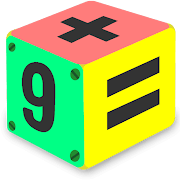Math Puzzles game - Brain Training Math Games Free

Best Math Games for kids teens & adults! Fun math brain puzzles game free

4.25 PUZZLE GAME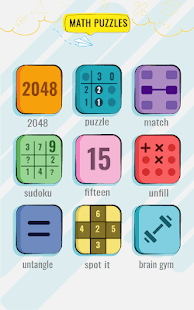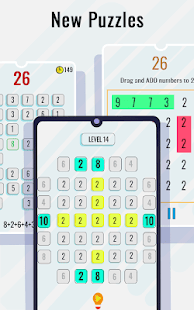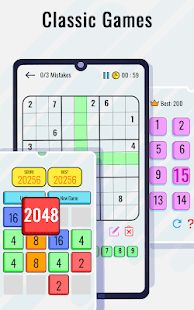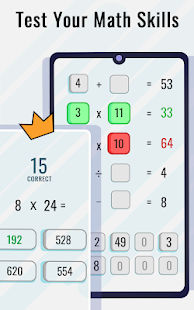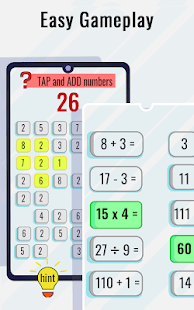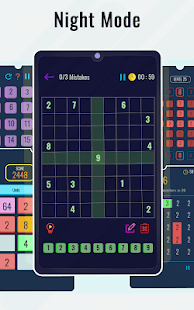Best maths puzzle game to train your brain & is designed for all ages including kids, girls and boys, adults. You will train your brain to test mind, skill and speed. Math games are a brain training game 🧠🧩.Excellent educational game for learning mathematics for kids and adults of all ages. Solve challenging and cool math puzzles game today on android and be Math Master by doing brain maths! Math games for adults is the best available maths puzzle game or maths puzzle app.

Math games for kids, teens & adults, not only train your brain, but it will also reveal the true potential of your brain. This mathematical game (math puzzle 🧩) helps you solve a lot of interesting mathematical games. Brain games help you practice different mental skills: memory, attention, speed, reaction, concentration, logic, mathematics, maths puzzle game, brain maths, time tables, and more math quiz. math think fast - matching puzzle mathematics game are best for brain training. Great math puzzle and riddle game for all

Benefits of Mathematical puzzles game & mental arithmetic puzzle?

💯12 mini Math games improve attention & focusing on logical maths puzzle game
💯Math Brain games develop memory power and perception abilities
💯Logical puzzles help to manage stress control in an entertaining way
💯Fun Math games to play with friends & mental maths

Math puzzles is handpicked by experts of Math Games, Brain games, Educational games, Number games & logical puzzles all in one collection. Cool math games teach you multiply, divide, subtract and square, math puzzle and riddle game. math puzzles for free is for all ages.

Math Games Features (mathematical puzzle)

🧠 Addictive Classic puzzle maths number game
🧠 Classic math puzzle 15 game and maths puzzle app
🧠 Enhance your brain with cool math games, play and be a mathematics prodigy
🧠 Math piece puzzle to train your brain with new Maths puzzle app.
🧠 Brain Games with Math Tricks and Cool Maths
🧠 Increase your brainpower with free Maths puzzle app
🧠 Fast math workout, maths puzzle game, Mental math games for kids & adults
🧠 All in one Math app with mini Maths game for adults & math puzzles
🧠 Best fun Maths play game with dark mode and arithmetic puzzle
🧠 Cool math Games app is a brain-training arithmetic puzzle
🧠 Math games is a brain gym to reach their full potential
🧠 Brain Maths is an excellent math games for adults too
🧠 Maths Tricks to learn multiplication, fractions, addition, geometry, algebra, problem solving

Increase the result and feel confident in the standard tests: iq test, GRE, GMAT, ACT, MCAT, etc.
Math games for adults and kids can be played offline and is free to use maths puzzle games. And having puzzles with solution and hint. This maths puzzle games contains best arithmetic puzzle, brain maths, in a fun math playground. Maths puzzle app is easy to use. Maths puzzle app is completely free and contains puzzles with solution and hint. Brain maths is for everyone

Brain games is based on principles of cognitive psychology to help you practice different mental skill: memory, attention, speed, reaction, concentration, logic and more in Maths puzzle app. Download now math puzzle and riddle game and see the leaning. Arithmetic puzzle is free math with math exercises. Improve your brain maths with the math puzzle workout given in this math playground.

Top Math Games free! Addition, Subtraction, Multiplication & Division! Practice math while having fun with the best math games!➕➖✖️➗ ✔️. Download now math puzzles for free. And find those puzzles with solution and hint.

For all ages: games for kids, for parents, for teens and for everybody, arithmetic puzzle!
Improve your math skills with this android application on Google play today!

Added 3 new Math Games - Math piece puzzle, Calcudoku, Number Merge puzzle Added new languages - Spanish, Portuguese, Deutsche, Italiano, French, Russian, Indonesian, Vietnamese, Malay Total 12 math games with 300 new levels All in one Math Games Please rate us if you like the game

Other versions

2.3 released on 18 May 2020 (616 days ago)

Details and Recent Ratings

Size: 0 MB
Version: 3.0 by GunjanApps Studios
Updated: 20 July 2020 (553 days ago)
Released: 17 May 2020
Installations: more than 50 000
5 Stars: 154
4 Stars: 30
3 Stars: 0
2 Stars: 30
1 Star: 15

Similar apps

Android Game Categories
Android App Categories